## Introduction

The prospect of manipulating the valley degree of freedom in materials like AlAs1, silicon2,3,4 graphene5, MoS26,7,8,9, or silicene10 is stimulating considerable interest as it could lead to a new paradigm in technology exploiting valleys in addition to charge and spin: valleytronics. From the fundamental point of view, valley-physics provides new insight into fundamental questions such as whether or not electrons confined to two dimensions conduct electricity when the temperature approaches absolute zero, which remains a subject of intense research and controversy despite decades of research. In the 1970's, the scaling theory of conductance established that at T = 0 K, a noninteracting two-dimensional electron gas (2DEG) would be localised by any amount of disorder at sufficiently large scales11. This prediction was verified experimentally rapidly12 and shaped our understanding of the two-dimensional electron gas for the next two decades despite theoretical refinements taking into account electron-electron interaction predicting the possibility of delocalisation13,14,15,16,17,18. Valley degeneracy increases the effect of interactions and indeed, the 2DEG in silicon MOSFETs which possesses this degeneracy, was the system in which strong metallic behaviour was first observed19,20,21. The precise understanding of real systems is however complicated because valley degeneracy is accompanied by intervalley scattering and possibly valley splitting22,23,24,25,26,27. Both these mechanisms are predicted to weaken the delocalising effect of interactions by reducing the number of active interaction channels22. While it is now well established experimentally that lifting valley degeneracy can destroy the metallic behaviour28,29, less is known about the effect of intervalley scattering. In order to fully understand the role played by intervalley scattering, it is important to compare the behaviour of systems ranging from those with very weak intervalley scattering to strong intervalley scattering. However, previous studies in which the role of intervalley scattering on interactions has been analysed had been limited to systems where this scattering is only weak or moderate so that its effect on the metallic behavour is either absent or restricted to the lowest temperatures23,24,25. Here, we investigate two-dimensionnal electron gases confined in a SOI quantum well2,3,29 (See Methods) where intervalley scattering is an order of magnitude stronger than previously studied so that it modifies the interaction at temperatures up to about 10 K. In addition, compared to Si MOSFETs, interactions are particularly strong in SOI quantum wells because of the reduced dielectric constantof the surrounding SiO2. For example, the Wigner-Seitz parameter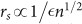at the density n = 3.9 × 1015 m−2 is rs = 8.2 compared to rs = 4.2 in MOSFETs (in Si MOSFETs,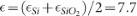while in SOI quantum wells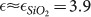). This allows the study of a regime where both interactions and temperatures are large while the system remains degenerate.

## Results

The longitudinal resistivity of our sample as function of temperature is displayed in Fig. 1a for different electron densities. An apparent metal-insulator transition is observed at nc ≈ 2.3 × 1015 m−2 where the resistivity is about 2 × h/e2. Deep in the metallic regime, the region of interest for the present study, the conductivity at B = 0 T increases linearly when temperature decreases (Figure 2). At n = 3.9 × 1015 m−2, where the mobility at T = 0.35 K is 0.3 m2/Vs, conductivity increases by 60% when temperature is decreased from 6 K to 0.35 K. At low fields and low temperatures, we attribute the sharp negative magneto-resistance seen in Fig. 1b to weak-localisation30.

In the Fermi Liquid theory, weak localisation and electron-electron interactions appear as additive corrections to the classical Drude conductivity σD18: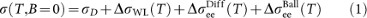where, ΔσWL is the weak localisation (WL) correction,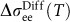is the electron interaction correction in the diffusive regime (kB/ < 1, where kB and are Boltzmann and reduced Planck constants and τ is the Drude scattering time) and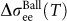is the electron interaction correction in the ballistic limit (kB/ > 1). Historically, the diffusive and ballistic interaction corrections had been thought to be governed by a different physics13,17. By studying intermediate temperatures (kB/ ~ 1) where both terms contribute, Zala, Narozhny and Aleiner (ZNA) established that they are governed by the same effect: the scattering off Friedel oscillations, which extend longer or shorter distances compared to the mean free path depending on the regime of interaction18. Interestingly, the ballistic contribution is predicted to be linear in temperature and might therefore explain the linear temperature dependence observed in Fig. 2. In units of the quantum conductance e2/h, this correction is expressed as18: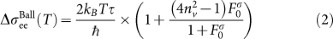where kB and h are Boltzmann and Plack constants, nv is the valley degeneracy and τ is obtained from the Drude conductivity σD = ne2τ/m* (e is the electron charge and m* their effective mass). We estimate τ to be 3.8 × 10−13 s by extrapolating the conductivity to T = 0 K as suggested in Ref. 18. The interaction parameter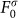is usually determined from the slope of the conductivity as function of temperature, assuming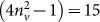due to the double valley degeneracy in (001) silicon quantum wells22. However, intervalley scattering mixes the valleys in real samples and reduces the number of diffusion channels from 15 to 3 for temperatures lower than T = /kBτ22. Here τ is the intervalley scattering time. It is therefore important to determine in which regime of intervalley scattering the sample is in.

Intervalley scattering time can be extracted from the weak localisation magneto-conductivity (MC)31,32. At low magnetic field and for strong intervalley scattering, the MC is that of a single valley system since the valleys are mixed at the time scale of the phase coherence time τφ. As the magnetic field is increased, the typical length of the trajectories contributing to the WL correction decreases so that the valley mixing along these trajectories decreases. It is suppressed for B Bv/2eDτ (Here D = 1/2vFτ is the diffusion coefficient where vF is the Fermi velocity). As a result, the system approaches the MC of a system with no intervalley scattering (i.e. two times that of a single valley system). We used a recent theory interpolating between these regimes32 to fit the experimental data. The two adjustable parameters were τ and τφ (See supplementary information for the code used).

The result of this procedure is displayed in Fig. 3. The theory describes perfectly the experimental data. We checked that the phase coherence time obtained by fitting the MC with the Hikami formula30 is consistent with that obtained with the equations of Ref. 32 (see Fig. 3). The Hikami formula is known to provide good estimates of τφ even in systems with intervalley scattering if sufficiently low field is used for the fitting32. The phase breaking time follows the T−1 temperature dependence expected for electron-electron interactions33,34. The intervalley scattering time is found to be smaller than the phase coherence time in the entire temperature range confirming that intervalley scattering is strong in our sample. In addition, τ is temperature independent suggesting that it is governed by static disorder32.

Let us now determine the number of active interaction channels in our sample. From the measured value of τ we obtain T ≈ 7.5 K. This means that our 2DEG behaves like a single valley system from the point of view of interaction corrections to conductivity in the entire range of temperatures. In other words, the factor (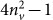) should be replaced by 3 in Eq. 2. By fitting the experimental conductivity to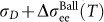we obtain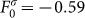and verify that the ballistic contribution of electron-electron interaction correction to conductivity describes our data very well (See Fig. 2). The value of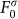seems very large compared to that obtained previously in Si MOSFETs for comparable electron density. However, we recall that in SOI, interactions are enhanced so that from the point of view of interactions, our system behaves like a usual MOSFETs of much lower electron density. At comparable value of rs,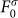is also of the order of −0.6 in usual Si MOSFETs (See Fig. 5b in Ref. 24). This confirms the consistency of the results obtained in the two systems. Here, we should stress that it is very surprising that the ballistic interaction correction alone can describe the conductivity. Indeed, given the measured value of τ, we have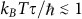and therefore the system is entering the diffusive regime. In addition, as we have already observed, WL produces a large MC and should therefore also contribute significantly to the temperature dependence of conductivity at B = 0 T. This paradox of the coexistence of weak localisation magneto-resistance and the absence of its temperature dependence at B = 0 T is evident in previously reported data35 but has remained largely overlooked although an interesting interpretation was suggested in Ref. 36. Following and extending the analysis of Ref. 36, we now show how the paradox is resolved in our case.

At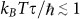all contributions to Eq. 1 have to be taken into account37,38. The contribution of weak localisation is readily known once the WL magneto-conductivity is analysed. It reads11: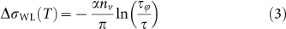where α = 0.5 in presence of strong intervalley scattering. This contribution is plotted as filled triangles in Fig. 2. As expected, weak localisation does indeed make a large negative contribution, especially at low temperatures. This contribution has to be cancelled by the diffusive interaction correction which is predicted to be13,18: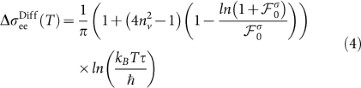Here again, the factor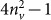has to be replaced by 3 in our case of strong intervalley scattering. We have used a different symbol for the interaction parameter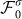to stress that there is no reason for it to be the same in the diffusive and ballistic regimes18,37,38,39. This contribution is plotted as open triangles in Figure 2 for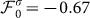while the sum of all three contributions is plotted as opened stars. As can be seen, the localising effect of WL can indeed be counterbalanced by the delocalising effect of diffusive electron-electron interactions. This is an important result of our study which explains the apparent paradox of a ballistic-like temperature dependence of the conductivity while the system is entering the diffusive regime of interactions. In addition to the conductivity at B = 0 T, ZNA theory makes a prediction for the temperature dependence of the Hall coefficient40. Figure 1c shows that in our case the Hall coefficient is surprisingly temperature independent. This observation is in fact very well described by the theory which predicts the absence of temperature dependence of the Hall coefficient for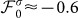(see Fig. 2 and its caption in Ref. 40). The theory also provides a prediction for the phase breaking time which can be obtained recursively as described in Ref. 34. The solid blue line in Fig. 3 represents this prediction and shows that the theory agrees well with our data. All transport coefficients and the phase coherence time are therefore described consistently and we conclude that the theory captures the observed phenomenology.

## Discussion

The cancellation of two quantum effects is a surprising observation and it is interesting to consider to what extent our experimental conditions are special in enabling us to observe the cancellation of weak localisation and diffusive interaction correction. Other authors have already reported35 on the co-existence of linear temperature dependence of conductivity at zero field and the weak localisation magneto-resistance (i.e. the evidence of the cancellation). We have performed a similar analysis on the data from sample Si15 published in Ref. 35. The result is displayed in Fig. 4. Although intervalley scattering is weak in this sample (we find T ≈ 80 mK) and therefore all 15 multiplets contribute to the transport, we observe the cancellation. As expected from the lower interaction in Si MOSFETs, the interaction parameter obtained in Si15 (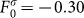and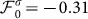) is smaller than in our sample for a comparable electron density. The values of interaction parameter are comparable to those obtained previously for similar values of rs24. In addition, a similar cancellation has also been reported in two-dimensional hole gases in SiGe, a single valley system36. In SiGe, the cancellation is found for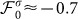, comparable to our observation. This confirms the consistency of our analysis since the cancellation should occur for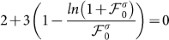in both systems which are single valley from the point of view of quantum corrections. The cancellation is therefore observed in a wide variety of samples with different strength of intervalley scattering and host material and we conclude that although it is not a universal behaviour (see Ref. 39 for an illustrative counter example), it is also not an extremely rare effect. Let us now consider the density dependence of the cancellation. We found that the cancellation is still observed at n = 4.9 × 1015 cm−2 (Fig. 2). We interpret this as resulting from the weak density dependence of the interaction parameter. Since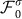weakly depends on the density (We found τ = 10−12 s,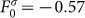and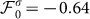at n = 4.9 × 1015 cm−2),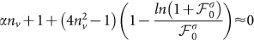over a wide range of densities.

Before concluding we note that in the theoretical expressions of the ZNA theory, equation 2 contains two dimensionless transition functions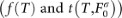to refine the description of the crossover between diffusive and ballistic limits18. In the analysis presented, we have followed the prescription of ZNA which states that “they can be neglected for all practical purposes”18, as also followed successfully by other experimental studies36,38 and this has enabled us to capture the essential physics of the system. However, when these functions are included, we find that we are not able to match the theory to our data. At present, the extent to which neglecting these functions is justified theoretically remains unclear and an analysis of sufficient detail required for a meaningful treatment is beyond the scope of the present work.